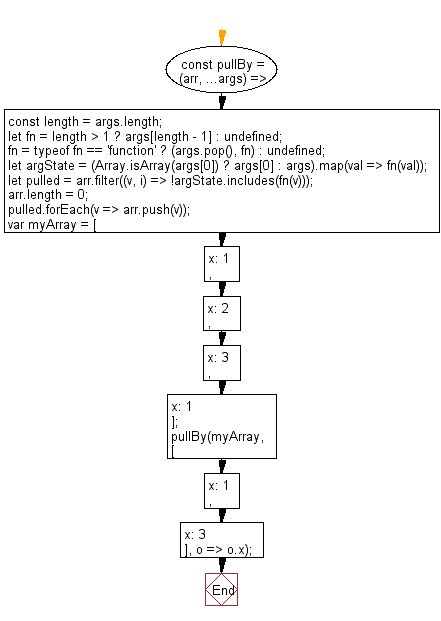# JavaScript: Mutate the original array to filter out the values specified, based on a given iterator function

## JavaScript fundamental (ES6 Syntax): Exercise-160 with Solution

Write a JavaScript program to mutate the original array to filter out the values specified, based on a given iterator function.

• Check if the last argument provided is a function.
• Use Array.prototype.map() to apply the iterator function fn to all array elements.
• Use Array.prototype.filter() and Array.prototype.includes() to pull out the values that are not needed.
• Set Array.prototype.length to mutate the passed in an array by resetting its length to 0.
• Use Array.prototype.push() to re-populate it with only the pulled values.

Sample Solution:

JavaScript Code:

``````//#Source https://bit.ly/2neWfJ2
const pullBy = (arr, ...args) => {
const length = args.length;
let fn = length > 1 ? args[length - 1] : undefined;
fn = typeof fn == 'function' ? (args.pop(), fn) : undefined;
let argState = (Array.isArray(args) ? args : args).map(val => fn(val));
let pulled = arr.filter((v, i) => !argState.includes(fn(v)));
arr.length = 0;
pulled.forEach(v => arr.push(v));
};

var myArray = [{ x: 1 }, { x: 2 }, { x: 3 }, { x: 1 }];

pullBy(myArray, [{ x: 1 }, { x: 3 }], o => o.x);

console.log(myArray);
```
```

Sample Output:

```[{"x":2}]
```

Pictorial Presentation:Flowchart:Live Demo:

See the Pen javascript-basic-exercise-160-1 by w3resource (@w3resource) on CodePen.

Improve this sample solution and post your code through Disqus

What is the difficulty level of this exercise?

Test your Programming skills with w3resource's quiz.

﻿